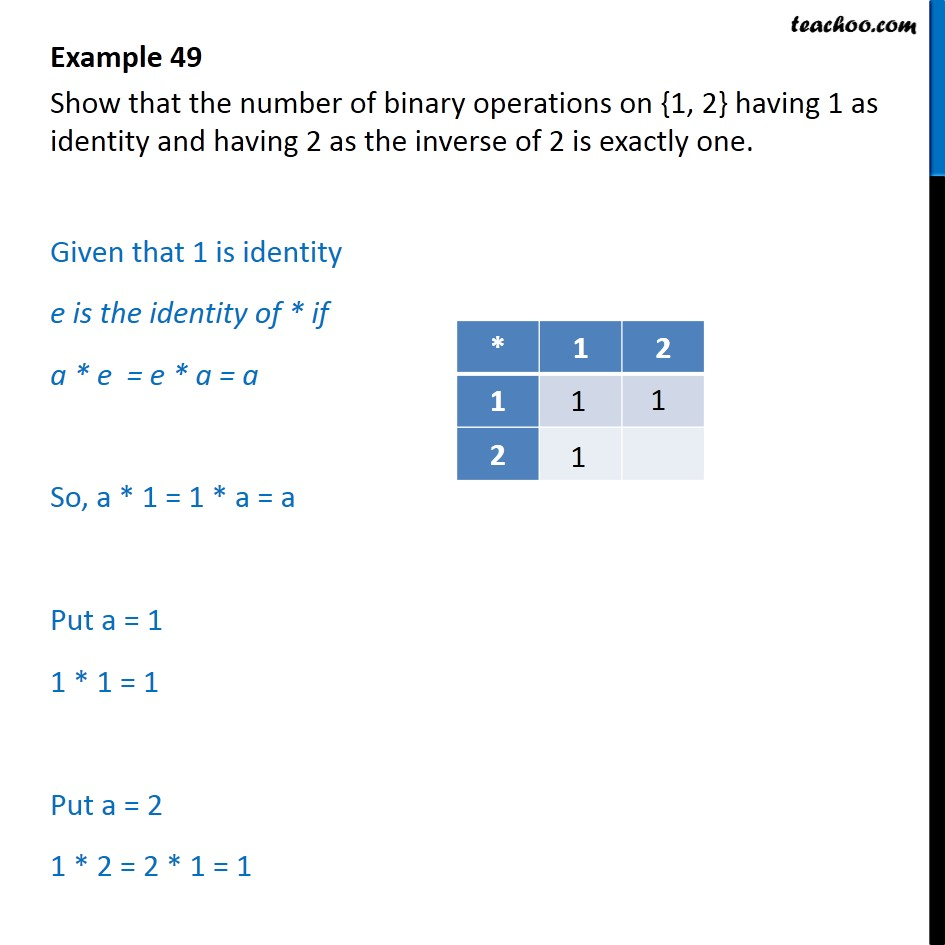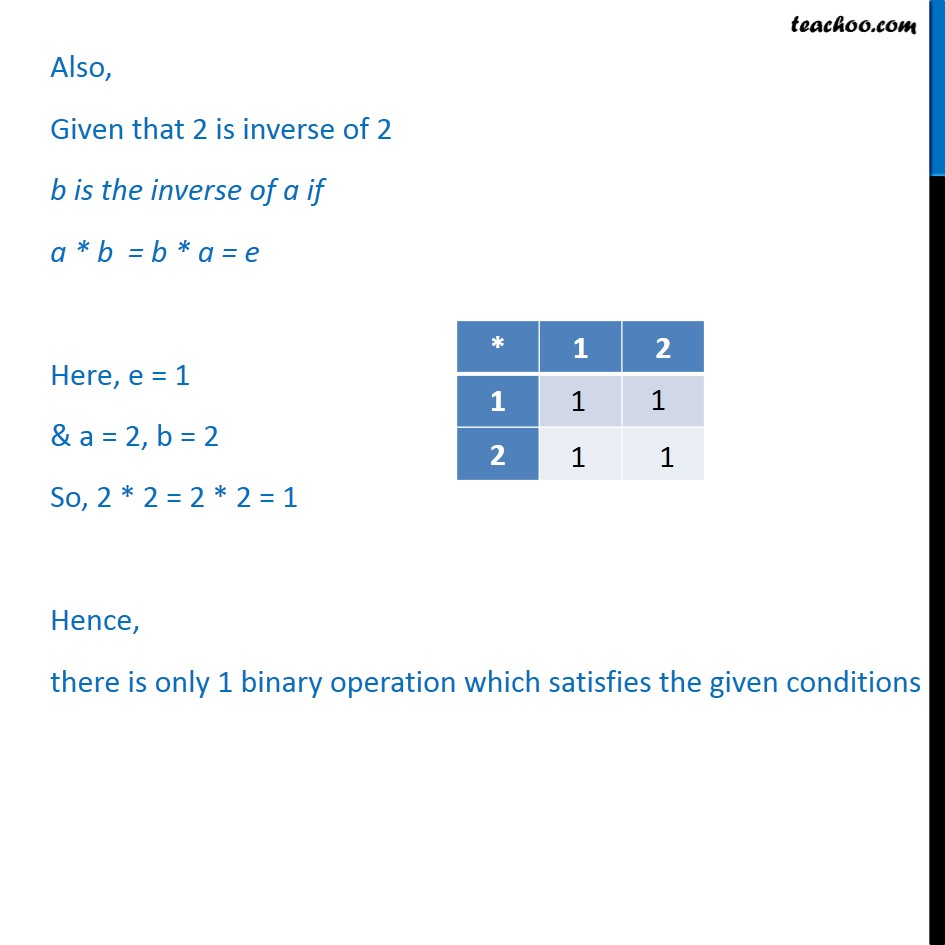Binary operations: Inverse

Chapter 1 Class 12 Relation and Functions
Concept wiseIntroducing your new favourite teacher - Teachoo Black, at only ₹83 per month

### Transcript

Example 49 Show that the number of binary operations on {1, 2} having 1 as identity and having 2 as the inverse of 2 is exactly one. Given that 1 is identity e is the identity of * if a * e = e * a = a So, a * 1 = 1 * a = a Put a = 1 1 * 1 = 1 Put a = 2 1 * 2 = 2 * 1 = 1 Also, Given that 2 is inverse of 2 b is the inverse of a if a * b = b * a = e Here, e = 1 & a = 2, b = 2 So, 2 * 2 = 2 * 2 = 1 Hence, there is only 1 binary operation which satisfies the given conditions